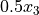# Problem modification and re-solving

After invoking `KN_solve()` to perform an initial solve of the model, you may wish to make some small modifications to your model and then re-solve. This section outlines the modifications that can be made to an existing model after an initial solve, which allow for re-solving the existing model using the same Knitro solver instance.

Generally, as long as no structural changes beyond linear changes are made to the model, `KN_solve()` can be called in succession to re-solve a model after modifications. For example, user options, variable bounds, and constraint bounds can be changed between calls to `KN_solve()`, without having to first call `KN_free()` and reload the model from scratch. In addition, constant and linear structures in the model may be added, deleted, or changed without having to reconstruct the model. More extensive additions or changes to the model (such as adding quadratic structures or callbacks) require freeing the existing Knitro solver object and rebuilding the model from scratch.

More specifically, the following API functions may be used to modify a problem before re-solving.

There are a few important points to note regarding problem modification.

1. The constant and linear structural changes in the model implemented through `KN_add_*()`, `KN_del_*()`, and `KN_chg_*()` functions are not actually updated until `KN_solve()` is called. Therefore, any API functions that retrieve these problem structures will not reflect any new changes made since the last call to `KN_solve()`. In contrast, typically model changes implemented through `KN_set_*()` functions (such as setting variable or constraint bounds) are updated immediately.

2. API functions that delete problem structure can only be used to delete structure that existed in the model prior to the last call to `KN_solve()`. Any new structure added since the last call to `KN_solve()` cannot be deleted. In practice, there would be no reason to delete such structure, since you can just avoid adding it in the first place if it is not wanted.

3. API functions for changing structures are just shorthand for deleting and then adding new structures. For example, if you want to change an existing linear constraint coefficient, calling `KN_chg_con_linear_struct()` is identical to calling `KN_del_con_linear_struct()` followed by `KN_add_con_linear_struct()` on that coefficient.

4. Although there is not currently an API function to directly remove linear constraints (Knitro API functions work on structures, rather than constraints), a linear constraint will effectively be removed by calling the API functions `KN_del_con_constants()` and `KN_del_con_linear_struct()` to remove all the constant and linear structures in the constraint, and removing any existing constraint bounds (by setting the lower constraint bound to `-KN_INFINITY` and the upper constraint bound to `KN_INFINITY` if necessary).

For more details on the API functions described above, please see the callable library API section in the Reference Manual Callable library API reference. Below we provide a simple example, demonstrating how to modify and then re-solve a problem using the same problem context structure. More examples may be found in the Knitro distribution `examples/C` directory.

## Example

Consider the following nonlinear optimization problem from the Hock and Schittkowski test set provided as example `examples/C/exampleNLP2.c`.

```max   x0*x1*x2*x3         (obj)
s.t.  x0^3 + x1^2 = 1     (c0)
x0^2*x3 - x2 = 0    (c1)
x3^2 - x1 = 0       (c2)
```

In `examples/C/exampleNLP2Resolve.c` and below, we show how to modify this problem by adding, deleting, or changing linear structures in the model, and then re-solve using the same Knitro solver instance.

Note

The Knitro distribution comes with several C language programs in the directory examples/C. The instructions in `examples/C/README.txt` explain how to compile and run the examples.

We will not repeat the code for solving the original model above. This is covered in the example from section Derivatives and in file `examples/C/exampleNLP2.c`.

In the code segments below, we show the modifications made after the initial call to `KN_solve()`.

First, after the initial solve, we add a new linear termto (c2), and add a new linear inequality constraint (c3) so the model becomes:

```max   x0*x1*x2*x3             (obj)
s.t.  x0^3 + x1^2 = 1         (c0)
x0^2*x3 - x2 = 0        (c1)
x3^2 - x1 + 0.5x3 = 0   (c2)
x1 + 2x2 + x3 <= 2.5    (c3)
```

Then it is re-solved using the same kc structure.

```/*... Solve the initial problem. ...*/
nStatus = KN_solve (kc);

/*=============== MODIFY PROBLEM AND RE-SOLVE ===========*/

/** Add 0.5x3 linear term to c2 */
nVar = 3;
dCoef = 0.5;
error = KN_add_con_linear_struct_one (kc, 1, 2,
&nVar,
&dCoef);
if (error) exit(-1);

/** Now add a new linear constraint
*    x1 + 2x2 + x3 <= 2.5 (c3)
*  and re-solve. */
if (error) exit(-1);
error = KN_set_con_upbnd(kc, cIndNew, 2.5);
if (error) exit(-1);
/** Coefficients for 3 linear terms */
lconNewIndexVars = 1; lconNewCoefs = 1.0;
lconNewIndexVars = 2; lconNewCoefs = 2.0;
lconNewIndexVars = 3; lconNewCoefs = 1.0;
error = KN_add_con_linear_struct_one (kc, 3, cIndNew,
lconNewIndexVars,
lconNewCoefs);
if (error) exit(-1);

/** Tell Knitro to try a "warm-start" since it is starting from the solution
*  of the previous solve, which may be a good initial point for the solution
*  of the slightly modified problem. */
error = KN_set_int_param (kc, KN_PARAM_STRAT_WARM_START, KN_STRAT_WARM_START_YES);

/** Re-solve the modified problem. */
nStatus = KN_solve (kc);
```

Next we remove the linear term 0.5x3 from (c2), and change the linear term x1 in c(3) to be 3.5x1 so the model becomes

```max   x0*x1*x2*x3             (obj)
s.t.  x0^3 + x1^2 = 1         (c0)
x0^2*x3 - x2 = 0        (c1)
x3^2 - x1 = 0           (c2)
3.5x1 + 2x2 + x3 <= 2.5 (c3)
```
```/*=============== MODIFY PROBLEM AND RE-SOLVE AGAIN ===========*/

/** Delete 0.5x3 linear term from c2 */
nVar = 3;
error = KN_del_con_linear_struct_one (kc, 1, 2,
&nVar);
if (error) exit(-1);

/** Change x1 linear term in c3 to 3.5*x1 */
nVar = 1;
dCoef = 3.5;
error = KN_chg_con_linear_struct_one (kc, 1, 3,
&nVar, &dCoef);
if (error) exit(-1);

/** Re-solve the modified problem again. */
nStatus = KN_solve (kc);
```